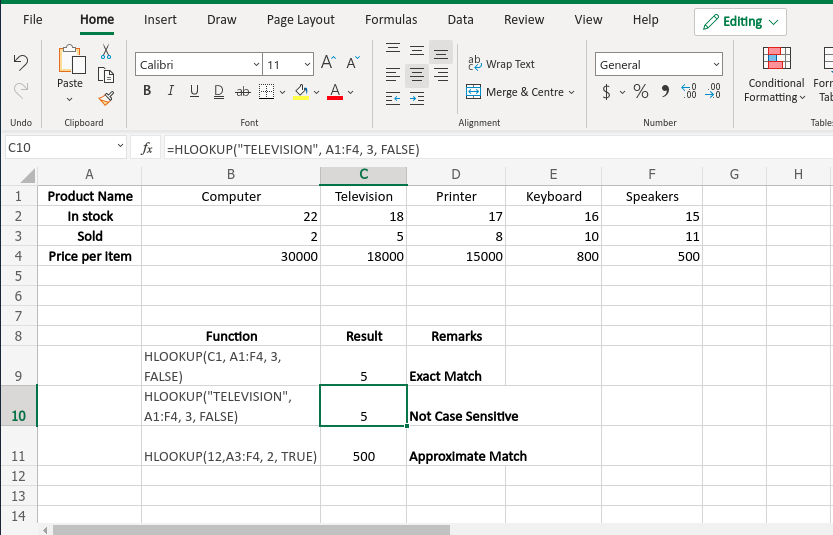# HLOOKUP Function in Excel With Examples

• Last Updated : 24 May, 2021

Excel has several functions to search data from a table. HLOOKUP() function is one of them. The ‘H’ in the HLOOKUP() function stands for ‘horizontal’. This function generally helps to find a value from a row of an array or table. For this function to work the lookup value must be in the first row of the table or array. HLOOKUP() moves downward searches for the required output. Like VLOOKUP() this function also supports exact and approximate match.

HLOOKUP() function is used when the user tries to find a value in a table by matching a lookup value in the first row of the table. Generally, the user wants an exact match. This function searches for a value horizontally as its name means.

Syntax:

`=HLOOKUP(lookup_value, table_array, row_index_num, [range_lookup])`

Here [range_lookup] argument is optional.

So, basically HLOOKUP() takes four arguments as input and returns the desired output.

Arguments:

• lookup_value (Required): This is the value( maybe a value, a reference, or a text string) that HLOOKUP() function tries to match in the first row of the table. This argument must be provided by the user.
• table_array (Required): This is the table or the reference of a table where the HLOOKUP() function tries to match the lookup_value and searches for the output. Also, this argument must be provided by the user.
• row_index_num (Required): This is the row number where the HLOOKUP() function searches for the output. This argument should be provided by the user. This value cannot be less than 1 or greater than the number of rows in the table_array. In both cases, this function shows an error(#VALUE! Or #REF!).
• [range_lookup] (Optional): This is an optional argument. It contains a logical value(TRUE or FALSE) by which HLOOKUP() can understand if the user wants an approximate or an exact match. This value is TRUE by default(If the user does not provide any value). TRUE denotes an approximate match and FALSE denotes an exact match.

Note: If [range_lookup] value is FALSE and the user wants to match a text partially, then wildcards(‘*’ or ‘?’) can be used. An asterisk matches a sequence and question mark matches a single character. And this function is not case-sensitive.

Return Value: HLOOKUP() first matches the lookup value in the first column of the table and then moves downward to search the output value. After all, it returns that output value.

Example:

The list below is taken for the example:

In the above list HLOOKUP() function is applied:

Output:Output Screenshot

My Personal Notes arrow_drop_up